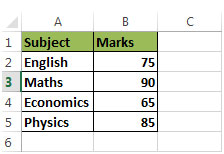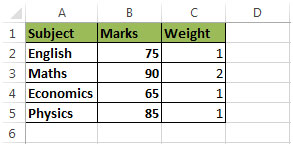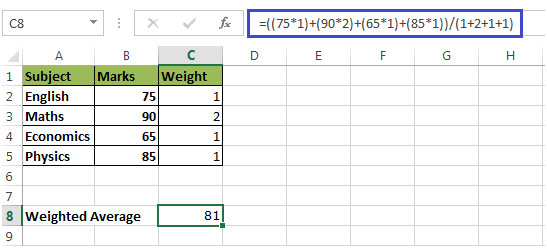# Calculating Weighted Average in Microsoft Excel

The concept of average comes from mathematics. Average can be defined as the result that we obtain after adding several quantities and then dividing this total by the number of quantities.

The weighted mean is similar to the arithmetic mean where instead of each of the data points contributing equally to the final average, some data points contribute more than others.

If all the weights are equal, then the weighted mean remains same as the arithmetic mean.
Let us understand more with an example:

We want to calculate the average of Marks of a Student in four subjects. In column A, we have Subject & in column B we have Marks• We will add the numbers & then divide the result by number of subjects
• (75 + 90 + 65 + 85) / 4 = 78.5
• This is the un-weighted average because in this scenario, we have assigned same priority to each number.

Weighted Average is a type of average where item of the weight is also taken into account while finding the average,because this one element may contribute heavily to the final result than another element.
Now let us understand with another example

In column A, we have Subject & in column B, we have MarksLet us assume that a student want to get admission to Math college because of interest in Mathematics.The College team has realized that a higher percentage of students want to get admission in their college because of good Faculties available & their excellent track record for the Math subject.So, obviously the college will put more emphasis on the Math marks and hence, they have come up with an idea of assigning weight to each subject as follows:• This shows the college lays more emphasis on the marks of Math as compared to marks of other subjects
• If we multiply Marks with Weight i.e. (Marks x Weight) we get:((75 x 1) + (90 x 2) + (65 x 1) + (85 x 1)) / (1 + 2 + 1 + 1)
• This will come out to be 81• Weighted Average (81) is higher than the Un-weighted Average (78.5)
• So, in this case, the Math’s marks have contributed more to the final output than any other element.
• With the above shown example, we have understood the difference between Weighted & Un-weighted Average.

1.This is the best example a student can imagine and learn the differences between arithmetic and weighted average.

2.